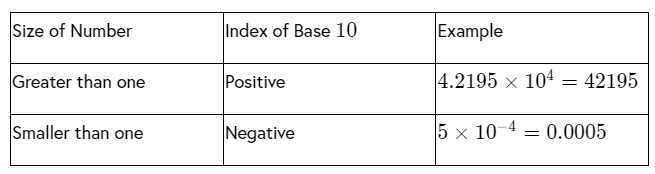# Introduction to law of indices

Last updated: September 21, 2021

## What are indices?

Indices are a convenient tool in mathematics to compactly denote taking power or roots of a number. Taking power is simply a case of repeated multiplication of a number. Whereas taking root is equivalent to a fractional power of the number. Therefore, it is important to clearly understand the concepts as well as the law of indices.

Indices are helpful in a computer game, physics, pH and Richter measuring scales and many other subjects.

## E1.7A: Understand the meaning of indices (fractional, negative and zero), and use the rules of indices.

### Negative Indices

Negative indices are exponents or powers that have a minus sign in front of them.

Consider an example, $$\frac{a^{2}}{a^{6}}=a^{2-6}=a^{-4}$$

Therefore, $$\frac{a^{2}}{a^{6}}=\frac{1}{a^{4}}$$

So, negative indices can be written as a fraction.

In general, $$x^{-m}=\frac{1}{x^{m}}$$

### Fractional Indices

In fractional indices, the bottom number in the fraction stands for the type of the root.

Consider the example, $$x^{\frac{1}{3}}$$ denotes a cube root of $$3$$, which can be written as $$\sqrt{x}$$.

In general, $$x^{\frac{1}{m}}=\sqrt[m]{x}$$

The number on the top of the fractional powers gives the power of the root.

Consider the example, $$x^{\frac{2}{3}}=\left(\sqrt{x} \right )^{2}$$

In general, $$x^{\frac{m}{n}}=\left(\sqrt[n]{x} \right )^{m}$$

Zero indices

The second law of indices is $$\frac{x^{m}}{x^{n}}=x^{m-n}$$

It helps to prove anything to power of zero is equal to $$1$$.

Anything divided by itself equals one. So, $$\frac{x^{3}}{x^{3}}=1$$

### Worked examples

Example 1: Write the numerical value of $$2^{-3}$$, $$\frac{1}{3^{-2}}$$, $$\frac{2}{5^{-2}}$$ and $$64\times {4}^{-2}$$.

Step 1: Recall the general form of negative indices and fractional indices.

$$x^{-m}=\frac{1}{x^{m}}$$ and  $$x^{\frac{m}{n}}=\left(\sqrt[n]{x} \right )^{m}$$

Step 2: Using the general form of negative indices and fractional indices, write the numerical value of $$2^{-3}$$

The numerical value of $$2^{-3}$$ is as follows:

$$2^{-3}=\frac{1}{2^{3}} = \frac{1}{8}$$

Step 3: Using the general form of negative indices and fractional indices, write the numerical value of $$\frac{1}{3^{-2}}$$.

The numerical value of $$\frac{1}{3^{-2}}$$ is as follows:

$$\frac{1}{3^{-2}}=3^{2}=9$$

Step 4: Using the general form of negative indices and fractional indices, write the numerical value of $$\frac{2}{5^{-2}}$$.

The numerical value of $$\frac{2}{5^{-2}}$$ is as follows:

$$\frac{2}{5^{-2}}=2\times 5^{2}$$

$$2\times (25)=50$$

Step 5: Using the general form of negative indices and fractional indices, write the numerical value of $$64\times {4}^{-2}$$.

The numerical value of $$64\times {4}^{-2}$$ is as follows:

$$64\times {4}^{-2}=64\times \frac{1}{4^{2}}$$

$$\frac{64}{16}=4$$

Example 2: Write the numerical value of $$20^{0}$$, $$\left(\frac{3}{4} \right )^{0}$$, $$4x^{0}+8y^{0}-{z}^{0}$$ and $$6\times {99}^{0}$$.

Step 1: Recall the zero indices.

The zero indices are $$a^{0}=1$$

Step 2: Use the zero indices to write the numerical value of $$20^{0}$$.

The numerical value of $$20^{0}$$ is as follows:

$$20^{0}=1$$

Step 3: Use the zero indices to write the numerical value of $$\left(\frac{3}{4} \right )^{0}$$.

The numerical value of $$\left(\frac{3}{4} \right )^{0}$$ is as follows:

$$\left(\frac{3}{4} \right )^{0}=1$$

Step 4: Use the zero indices to write the numerical value of $$4x^{0}+8y^{0}-{z}^{0}$$.

The numerical value of $$4x^{0}+8y^{0}-{3z}^{0}$$ is as follows:

$$4x^{0}+8y^{0}-{z}^{0}=\left(4\times 1 \right )+\left(8\times 1 \right )-1$$

$$4+8-1=11$$

Step 5: Use the zero indices to write the numerical value of $$6\times {99}^{0}$$.

The numerical value $$6\times {99}^{0}$$ is as follows:

$$6\times {99}^{0}=\left(6\times 1 \right )=6$$

### E1.7B: Use the standard form $$A\times 10^{n}$$, where $$n$$ is a positive or negative integer, and $$1\leqslant A< 10$$.

If a number has a large value that is complex to write, it is written in the standard form of $$A\times 10^{n}$$, where $$n$$ is a positive or negative integer, and $$1\leqslant A< 10$$.

Some examples are as follows:### Worked example

Example 1: The distance between Mercury and the Sun is $$5.79\times {10}^{7}\;\text{km}$$, and the distance between Venus and the Sun is $$10.8\times {10}^{7}\;\text{km}$$. Find the distance between Mercury and Venus.

Step 1: Write the given values.

The distance between Mercury and the Sun is $$5.79\times {10}^{7}\;\text{km}$$.

The distance between Venus and the Sun is $$10.8\times {10}^{7}\;\text{km}$$

Step 2: Sketch the orbits.$$\text{Minimum distance}=\left(10.8\times {10}^{7} \right )-\left(5.79\times {10}^{7} \right )$$

Step 3: Find the distance between Mercury and Venus.

$$\text{Minimum distance}=\left(10.8\times {10}^{7} \right )-\left(5.79\times {10}^{7} \right )$$

$$\left(10.8-5.79 \right )\times {10}^{7}=5.01\times {10}^{7}\;\text{ km}$$.

So, the distance between Mercury and Venus is $$5.01\times {10}^{7}\;\text{km}$$ or $$50100000\;\text{km}$$.